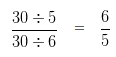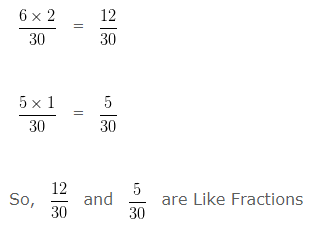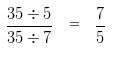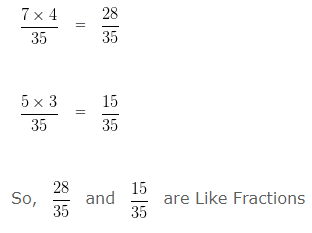# Like Fractions and Unlike Fractions

Like Fractions and Unlike Fractions, deals with various concepts which are as under:-

• Like Fractions
• Unlike Fractions
• Example of Like Fractions and Unlike Fractions
• Convert Unlike Fractions into Like Fractions

### Like Fractions

Like Fractions are the Fractions having same denominators.

For example 1/2, 3/2 and 5/2 are Like Fractions.

### Unlike Fractions

Unlike Fractions are the Fractions having different denominators.

For example 2/3, 3/5 and 4/7 are Unlike Fractions.

### Example of Like Fractions and Unlike Fractions

Example 1

Are the following fractions are Like Fractions or Unlike Fractions?

2/3, 4/3 and 5/3

Solution

In case of Like Fraction, the denominator of the fractions are the same.

While, In case of Unlike Fraction denominator of the fractions are not same.

In the present case, the given fractions are

2/3, 4/3 and 5/3

Since, the Denominator in the present cases is 3 , which is the same for all fractions, the given fractions are Like Fractions

Example 2

Are the following fractions are Like Fractions or Unlike Fractions?

4/5, 5/6 and 6/7

Solution

In case of Like Fraction, the denominator of the fractions are the same.

While, In case of Unlike Fraction denominator of the fractions are not same.

In the present case, the given fractions are

4/5, 5/6 and 6/7

Since the Denominator in the present cases are 5 , 6 , 7 which are not same. So, the given fractions are Unlike Fractions

### Convert Unlike Fractions to Like Fractions

Example 1

Convert 2/5 and 1/6 into Like Fractions.

Solution

Step1: Find LCM of the denominators.

LCM of 5 and 6 is 30

Step 2: Divide the LCM by the denominators of the given FractionsStep 3: Multiply the quotient by the numerator and keep denominator equal to the LCM. i.e.Example 2

Convert 4/5 and 3/7 into Like Fractions.

Solution

Step1: Find LCM of the denominators.

LCM of 5 and 7 is 35

Step 2: Divide the LCM by the denominators of the given FractionsStep 3: Multiply the quotient by the numerator and keep denominator equal to the LCM. i.e.